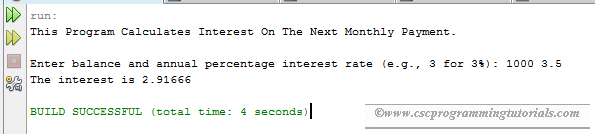# Exercise 2.20 - Java Interest Calculator

The program below is the solution to Liang's Introduction to Java Programming (9th Edition) Chapter 2 Exercise 2.20.

Question: If you know the balance and the annual percentage interest rate, you can compute the interest on the next monthly payment using the following formula:
interest = balance * (annualInterestRate /1200)
Write a program that reads the balance and the annual percentage interest rate and displays the interest for the next month.

```/**
*
* @Author: Aghatise Osazuwa
* Website: www.cscprogrammingtutorials.com
*
* Exercise 2.20 - Financial application: calculate interest
*
*/

import java.util.Scanner;

public class Ex02_20 {

public static void main(String[] args) {

//Display Program Information
System.out.println("This Program Calculates Interest On The Next Monthly"
+ " Payment.\n");

//create Scanner
Scanner input = new Scanner(System.in);

//prompt user to enter details
System.out.print("Enter balance and annual percentage interest rate "
+ "(e.g., 3 for 3%): ");
double balance = input.nextDouble();
double annualInterestRate = input.nextDouble();

//calculate interest using the formula interest = balance * (annualInterestRate / 1200)
double interest = balance * (annualInterestRate / 1200);
//format interest to five decimal places
interest = (int)(interest * 100000)/100000.0;

//display the result
System.out.println("The interest is " + interest + "\n");
}
}
```Program output
Click here to see other solutions to Introduction to Java Programming.